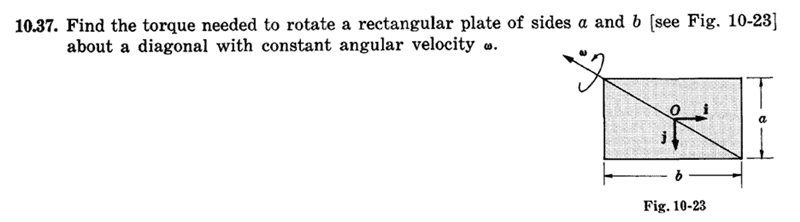# Conservation of angular momentum

• avito009

#### avito009

Angular momentum is the product of its moment of inertia and its angular velocity. So can we infer that since angular momentum is conserved then if an object has more moment of inertia then it will have lesser angular velocity and vice versa? Since from common sense we can make out that moment of inertia is rotational resistance and if this resistance is more the angular velocity will be less.

Yes, that's true. A very good example is the collapse to a neutron star. See e.g. here!

Angular momentum is the product of its moment of inertia and its angular velocity. So can we infer that since angular momentum is conserved...

To be more precise: angular momentum is only conserved when there is no net external torque.

Conserved when the net torque on it is zero. You may see the inertial moment play the similar role in rotation as the mass does in the linear motion.

Perhaps this funny and simple problem can enliven the conversation :)

Sorry in advance if that is inappropriateAngular momentum is the product of its moment of inertia and its angular velocity. So can we infer that since angular momentum is conserved then if an object has more moment of inertia then it will have lesser angular velocity and vice versa? Since from common sense we can make out that moment of inertia is rotational resistance and if this resistance is more the angular velocity will be less.
That is true if we are considering a particular object whose moment of inertia is changing over time (e.g. a skater pulling in her arms).

If we are considering two different objects then the principle does not hold. There is nothing that prevents one skater with a small moment of inertia from spinning slowly while another skater on the other end of the rink has a large moment of inertia and is spinning rapidly.

Angular momentum is the product of its moment of inertia and its angular velocity. So can we infer that since angular momentum is conserved then if an object has more moment of inertia then it will have lesser angular velocity and vice versa? Since from common sense we can make out that moment of inertia is rotational resistance and if this resistance is more the angular velocity will be less.

and on another thread you wrote..

If a top has angular momentum of 12 units and the Earth has angular momentum of 100. Does this mean that Earth is spinning faster than the top since it has more angular momentum? The answer is there at the back of my head but can't articulate it.

Both are wrong.

Angular Momentum is conserved (in systems that don't have an external torque applied). That doesn't mean Angular Momentum is the same for all systems. A car tyre has a much lower moment of inertia than the planet Earth yet it's rate of spin (angular velocity) is much higher. Perhaps many revolutions per second compared to one revolution per day.

Moment of inertia is similar to mass...

Linear... Force = mass * linear acceleration
Rotation... Torque = Moment of inertia * angular acceleration